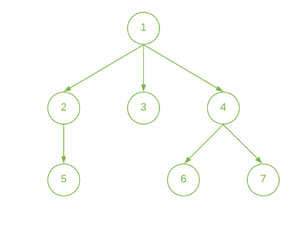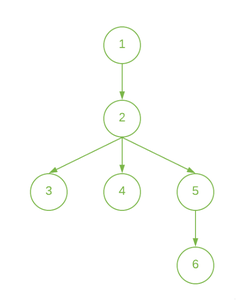# Farthest distance of a Node from each Node of a Tree

Given a Tree, the task is to find the farthest node from each node to another node in the given tree.
Examples

Input:Output: 2 3 3 3 4 4 4
Explanation:
Maximum Distance from Node 1 : 2 (Nodes {5, 6, 7} are at a distance 2)
Maximum Distance from Node 2 : 3 (Nodes {6, 7} are at a distance 3)
Maximum Distance from Node 3 : 3 (Nodes {5, 6, 7} are at a distance 3)
Maximum Distance from Node 4 : 3 (Node {5} is at a distance 3)
Maximum Distance from Node 5 : 4 (Nodes {6, 7} are at a distance 4)
Maximum Distance from Node 6 : 4 (Node {5} is at a distance 4)
Maximum Distance from Node 7 : 4 (Node {5} is at a distance 4)
Input:Output : 3 2 3 3 2 3

Approach:
Follow the steps below to solve the problem:

• Calculate the height of each node of the tree (Assuming the leaf nodes are at height 1) using DFS
• This gives the maximum distance from a Node to all Nodes present in its Subtree.. Store these heights.
• Now, perform DFS to calculate the maximum distance of a Node from all its ancestors. Store these distances.
• For each node, print the maximum of the two distances calculated.

Below is the implementation of the above approach:

## C++

 `// C++ Program to implement ` `// the above approach ` `#include ` `using` `namespace` `std; ` ` `  `#define maxN 100001 ` ` `  `// Adjacency List to store the graph ` `vector<``int``> adj[maxN]; ` ` `  `// Stores the height of each node ` `int` `height[maxN]; ` ` `  `// Stores the maximum distance of a ` `// node from its ancestors ` `int` `dist[maxN]; ` ` `  `// Function to add edge between ` `// two vertices ` `void` `addEdge(``int` `u, ``int` `v) ` `{ ` `    ``// Insert edge from u to v ` `    ``adj[u].push_back(v); ` ` `  `    ``// Insert edge from v to u ` `    ``adj[v].push_back(u); ` `} ` ` `  `// Function to calculate height of ` `// each Node ` `void` `dfs1(``int` `cur, ``int` `par) ` `{ ` `    ``// Iterate in the adjacency ` `    ``// list of the current node ` `    ``for` `(``auto` `u : adj[cur]) { ` ` `  `        ``if` `(u != par) { ` ` `  `            ``// Dfs for child node ` `            ``dfs1(u, cur); ` ` `  `            ``// Calculate height of nodes ` `            ``height[cur] ` `                ``= max(height[cur], height[u]); ` `        ``} ` `    ``} ` ` `  `    ``// Increase height ` `    ``height[cur] += 1; ` `} ` ` `  `// Function to calculate the maximum ` `// distance of a node from its ancestor ` `void` `dfs2(``int` `cur, ``int` `par) ` `{ ` `    ``int` `max1 = 0; ` `    ``int` `max2 = 0; ` ` `  `    ``// Iterate in the adjacency ` `    ``// list of the current node ` `    ``for` `(``auto` `u : adj[cur]) { ` ` `  `        ``if` `(u != par) { ` ` `  `            ``// Find two children ` `            ``// with maximum heights ` `            ``if` `(height[u] >= max1) { ` `                ``max2 = max1; ` `                ``max1 = height[u]; ` `            ``} ` `            ``else` `if` `(height[u] > max2) { ` `                ``max2 = height[u]; ` `            ``} ` `        ``} ` `    ``} ` ` `  `    ``int` `sum = 0; ` ` `  `    ``for` `(``auto` `u : adj[cur]) { ` `        ``if` `(u != par) { ` ` `  `            ``// Calculate the maximum distance ` `            ``// with ancestor for every node ` `            ``sum = ((max1 == height[u]) ? max2 : max1); ` ` `  `            ``if` `(max1 == height[u]) ` `                ``dist[u] ` `                    ``= 1 + max(1 + max2, dist[cur]); ` `            ``else` `                ``dist[u] ` `                    ``= 1 + max(1 + max1, dist[cur]); ` ` `  `            ``// Calculating for children ` `            ``dfs2(u, cur); ` `        ``} ` `    ``} ` `} ` ` `  `// Driver Code ` `int` `main() ` `{ ` `    ``int` `n = 6; ` ` `  `    ``addEdge(1, 2); ` `    ``addEdge(2, 3); ` `    ``addEdge(2, 4); ` `    ``addEdge(2, 5); ` `    ``addEdge(5, 6); ` ` `  `    ``// Calculate height of ` `    ``// nodes of the tree ` `    ``dfs1(1, 0); ` ` `  `    ``// Calculate the maximum ` `    ``// distance with ancestors ` `    ``dfs2(1, 0); ` ` `  `    ``// Print the maximum of the two ` `    ``// distances from each node ` `    ``for` `(``int` `i = 1; i <= n; i++) ` `        ``cout << (max(dist[i], height[i]) - 1) << ``" "``; ` ` `  `    ``return` `0; ` `} `

## Java

 `// Java program to implement ` `// the above approach ` `import` `java.util.*; ` ` `  `class` `GFG{ ` ` `  `static` `final` `int` `maxN = ``100001``; ` ` `  `// Adjacency List to store the graph ` `@SuppressWarnings``(``"unchecked"``) ` `static` `Vector []adj = ``new` `Vector[maxN]; ` ` `  `// Stores the height of each node ` `static` `int` `[]height = ``new` `int``[maxN]; ` ` `  `// Stores the maximum distance of a ` `// node from its ancestors ` `static` `int` `[]dist = ``new` `int``[maxN]; ` ` `  `// Function to add edge between ` `// two vertices ` `static` `void` `addEdge(``int` `u, ``int` `v) ` `{ ` `     `  `    ``// Insert edge from u to v ` `    ``adj[u].add(v); ` ` `  `    ``// Insert edge from v to u ` `    ``adj[v].add(u); ` `} ` ` `  `// Function to calculate height of ` `// each Node ` `static` `void` `dfs1(``int` `cur, ``int` `par) ` `{ ` `     `  `    ``// Iterate in the adjacency ` `    ``// list of the current node ` `    ``for``(``int` `u : adj[cur])  ` `    ``{ ` `        ``if` `(u != par) ` `        ``{ ` `             `  `            ``// Dfs for child node ` `            ``dfs1(u, cur); ` ` `  `            ``// Calculate height of nodes ` `            ``height[cur] = Math.max(height[cur], ` `                                   ``height[u]); ` `        ``} ` `    ``} ` ` `  `    ``// Increase height ` `    ``height[cur] += ``1``; ` `} ` ` `  `// Function to calculate the maximum ` `// distance of a node from its ancestor ` `static` `void` `dfs2(``int` `cur, ``int` `par) ` `{ ` `    ``int` `max1 = ``0``; ` `    ``int` `max2 = ``0``; ` ` `  `    ``// Iterate in the adjacency ` `    ``// list of the current node ` `    ``for``(``int` `u : adj[cur]) ` `    ``{ ` `        ``if` `(u != par) ` `        ``{ ` `             `  `            ``// Find two children ` `            ``// with maximum heights ` `            ``if` `(height[u] >= max1) ` `            ``{ ` `                ``max2 = max1; ` `                ``max1 = height[u]; ` `            ``} ` `            ``else` `if` `(height[u] > max2)  ` `            ``{ ` `                ``max2 = height[u]; ` `            ``} ` `        ``} ` `    ``} ` `    ``int` `sum = ``0``; ` ` `  `    ``for``(``int` `u : adj[cur]) ` `    ``{ ` `        ``if` `(u != par)  ` `        ``{ ` `             `  `            ``// Calculate the maximum distance ` `            ``// with ancestor for every node ` `            ``sum = ((max1 == height[u]) ?  ` `                    ``max2 : max1); ` ` `  `            ``if` `(max1 == height[u]) ` `                ``dist[u] = ``1` `+ Math.max(``1` `+ max2,  ` `                                       ``dist[cur]); ` `            ``else` `                ``dist[u] = ``1` `+ Math.max(``1` `+ max1, ` `                                       ``dist[cur]); ` ` `  `            ``// Calculating for children ` `            ``dfs2(u, cur); ` `        ``} ` `    ``} ` `} ` ` `  `// Driver Code ` `public` `static` `void` `main(String[] args) ` `{ ` `    ``int` `n = ``6``; ` `    ``for``(``int` `i = ``0``; i < adj.length; i++) ` `        ``adj[i] = ``new` `Vector(); ` `         `  `    ``addEdge(``1``, ``2``); ` `    ``addEdge(``2``, ``3``); ` `    ``addEdge(``2``, ``4``); ` `    ``addEdge(``2``, ``5``); ` `    ``addEdge(``5``, ``6``); ` ` `  `    ``// Calculate height of ` `    ``// nodes of the tree ` `    ``dfs1(``1``, ``0``); ` ` `  `    ``// Calculate the maximum ` `    ``// distance with ancestors ` `    ``dfs2(``1``, ``0``); ` ` `  `    ``// Print the maximum of the two ` `    ``// distances from each node ` `    ``for``(``int` `i = ``1``; i <= n; i++) ` `        ``System.out.print((Math.max(dist[i],  ` `                                 ``height[i]) - ``1``) + ``" "``); ` `} ` `} ` ` `  `// This code is contributed by 29AjayKumar `

## C#

 `// C# program to implement ` `// the above approach ` `using` `System; ` `using` `System.Collections.Generic; ` ` `  `class` `GFG{ ` ` `  `static` `readonly` `int` `maxN = 100001; ` ` `  `// Adjacency List to store the graph ` `static` `List<``int``> []adj = ``new` `List<``int``>[maxN]; ` ` `  `// Stores the height of each node ` `static` `int` `[]height = ``new` `int``[maxN]; ` ` `  `// Stores the maximum distance of a ` `// node from its ancestors ` `static` `int` `[]dist = ``new` `int``[maxN]; ` ` `  `// Function to add edge between ` `// two vertices ` `static` `void` `addEdge(``int` `u, ``int` `v) ` `{ ` `     `  `    ``// Insert edge from u to v ` `    ``adj[u].Add(v); ` ` `  `    ``// Insert edge from v to u ` `    ``adj[v].Add(u); ` `} ` ` `  `// Function to calculate height of ` `// each Node ` `static` `void` `dfs1(``int` `cur, ``int` `par) ` `{ ` `     `  `    ``// Iterate in the adjacency ` `    ``// list of the current node ` `    ``foreach``(``int` `u ``in` `adj[cur])  ` `    ``{ ` `        ``if` `(u != par) ` `        ``{ ` `             `  `            ``// Dfs for child node ` `            ``dfs1(u, cur); ` ` `  `            ``// Calculate height of nodes ` `            ``height[cur] = Math.Max(height[cur], ` `                                   ``height[u]); ` `        ``} ` `    ``} ` ` `  `    ``// Increase height ` `    ``height[cur] += 1; ` `} ` ` `  `// Function to calculate the maximum ` `// distance of a node from its ancestor ` `static` `void` `dfs2(``int` `cur, ``int` `par) ` `{ ` `    ``int` `max1 = 0; ` `    ``int` `max2 = 0; ` ` `  `    ``// Iterate in the adjacency ` `    ``// list of the current node ` `    ``foreach``(``int` `u ``in` `adj[cur]) ` `    ``{ ` `        ``if` `(u != par) ` `        ``{ ` `             `  `            ``// Find two children ` `            ``// with maximum heights ` `            ``if` `(height[u] >= max1) ` `            ``{ ` `                ``max2 = max1; ` `                ``max1 = height[u]; ` `            ``} ` `            ``else` `if` `(height[u] > max2)  ` `            ``{ ` `                ``max2 = height[u]; ` `            ``} ` `        ``} ` `    ``} ` `    ``int` `sum = 0; ` ` `  `    ``foreach``(``int` `u ``in` `adj[cur]) ` `    ``{ ` `        ``if` `(u != par)  ` `        ``{ ` `             `  `            ``// Calculate the maximum distance ` `            ``// with ancestor for every node ` `            ``sum = ((max1 == height[u]) ?  ` `                    ``max2 : max1); ` ` `  `            ``if` `(max1 == height[u]) ` `                ``dist[u] = 1 + Math.Max(1 + max2,  ` `                                       ``dist[cur]); ` `            ``else` `                ``dist[u] = 1 + Math.Max(1 + max1, ` `                                       ``dist[cur]); ` ` `  `            ``// Calculating for children ` `            ``dfs2(u, cur); ` `        ``} ` `    ``} ` `} ` ` `  `// Driver Code ` `public` `static` `void` `Main(String[] args) ` `{ ` `    ``int` `n = 6; ` `    ``for``(``int` `i = 0; i < adj.Length; i++) ` `        ``adj[i] = ``new` `List<``int``>(); ` `         `  `    ``addEdge(1, 2); ` `    ``addEdge(2, 3); ` `    ``addEdge(2, 4); ` `    ``addEdge(2, 5); ` `    ``addEdge(5, 6); ` ` `  `    ``// Calculate height of ` `    ``// nodes of the tree ` `    ``dfs1(1, 0); ` ` `  `    ``// Calculate the maximum ` `    ``// distance with ancestors ` `    ``dfs2(1, 0); ` ` `  `    ``// Print the maximum of the two ` `    ``// distances from each node ` `    ``for``(``int` `i = 1; i <= n; i++) ` `        ``Console.Write((Math.Max(dist[i],  ` `                                ``height[i]) - 1) + ``" "``); ` `} ` `} ` ` `  `// This code is contributed by Rohit_ranjan  `

Output:

```3 2 3 3 2 3
```

Time Complexity: O(V+E)
Auxiliary Space: O(N)

Attention reader! Don’t stop learning now. Get hold of all the important DSA concepts with the DSA Self Paced Course at a student-friendly price and become industry ready.

My Personal Notes arrow_drop_upCheck out this Author's contributed articles.

If you like GeeksforGeeks and would like to contribute, you can also write an article using contribute.geeksforgeeks.org or mail your article to contribute@geeksforgeeks.org. See your article appearing on the GeeksforGeeks main page and help other Geeks.

Please Improve this article if you find anything incorrect by clicking on the "Improve Article" button below.

Improved By : 29AjayKumar, Rohit_ranjan